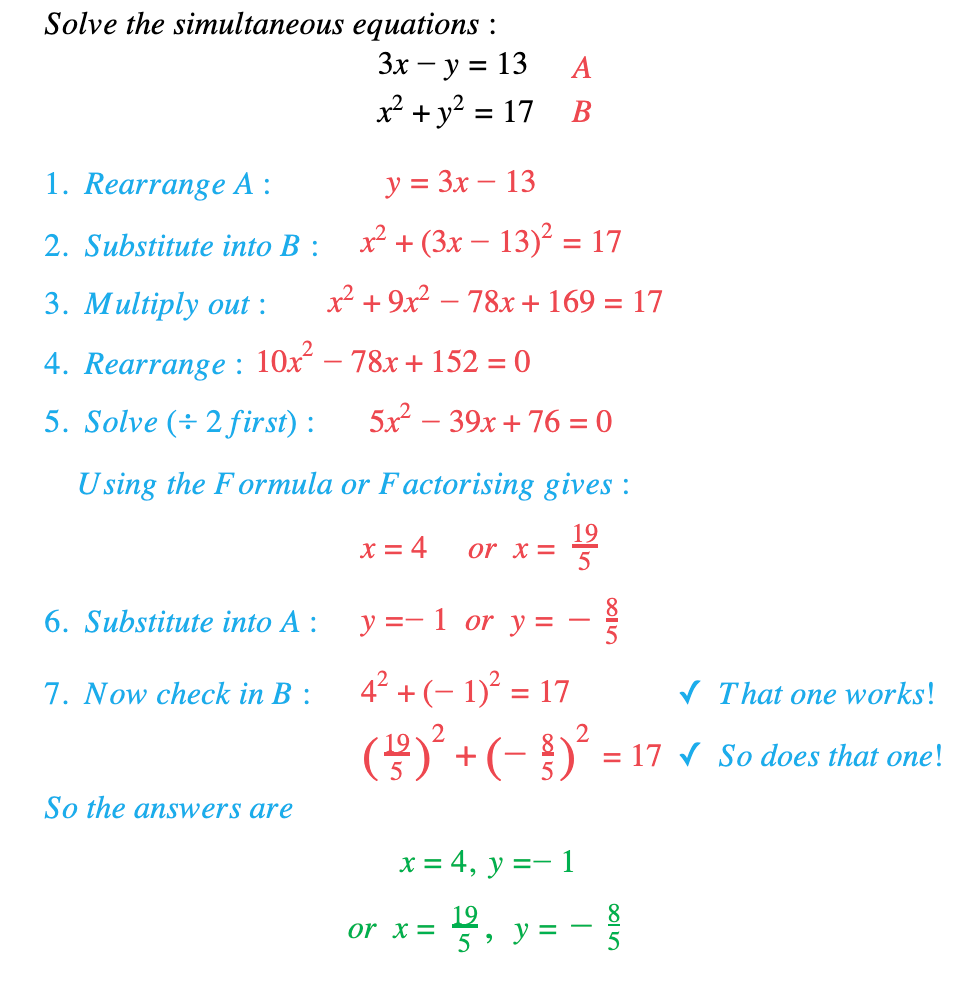# Edexcel IGCSE Maths 复习笔记 2.15.1 Quadratic Simultaneous Equations

Edexcel IGCSE Maths 复习笔记 2.15.1 Quadratic Simultaneous Equations

#### What are quadratic simultaneous equations?

• When there are two unknowns (say x and y) in a problem, we need two equations to be able to find them both: these are called Simultaneous Equations
• If there is an x2 or y2 in one of the equations then they are Quadratic (or Non-Linear) Simultaneous Equations

(They can be represented by a straight line and a curve on a graph – the two pairs of answers are the points of intersection of the line and the curve)

#### How do you solve quadratic simultaneous equations?

• This is called the Substitution Method:Label the equations A and B
1. Rearrange the linear equation to y = … (or x = …)
2. Substitute for y (or x) in the quadratic equation
3. Multiply out brackets
4. Rearrange to “quadratic = 0”
5. Solve using appropriate method (Factorisation or Formula)
6. Substitute back into the linear equation to find the other variable
7. Check your answer by substituting into the equation you didn’t use in 6

#### Exam Tip

If the resulting quadratic has a repeated root then the line is a tangent to the curve.If the resulting quadratic has no roots then the line does not intersect with the curve – or you have made a mistake!When giving your final answer, make sure you indicate which x and y values go together. If you don’t make this clear you can lose marks for an otherwise correct answer.

#### Worked Example